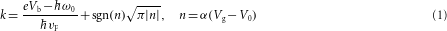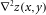## Main

Topographic corrugations and charge puddles in graphene are two of the most significant types of disorder in this new material. Topographic corrugations2,3,4, for example, have been suggested as a cause for the suppression of anticipated antilocalization5. Electron and hole puddles6 have similarly been blamed for obscuring universal conductivity in graphene7. These issues are part of a puzzle regarding the factors that limit graphene’s mobility8,9,10,11,12. In order for graphene to fulfil its promise as a next-generation nanodevice substrate it is important to understand the origin of the disorder and the influence it has on Dirac fermions. We have made new progress in this direction by using the techniques of scanning tunnelling microscopy (STM) and spectroscopy to simultaneously probe topographic and electronic disorder in graphene with an electron-density spatial resolution two orders of magnitude higher than previous scanning single-electron transistor microscopy measurements6.

Figure 1a shows the STM topography of a typical 30×30 nm2 area of a graphene monolayer on SiO2. We observe random corrugations with lateral dimension of a few nanometres and a vertical dimension of 1.5 Å (r.m.s.), probably due to roughness in the underlying SiO2 surface and/or intrinsic ripples of the graphene sheet2,3,4,13. STM imaging at the atomic scale clearly resolves the graphene honeycomb lattice on top of the broader surface corrugation all over the sample surface (inset).

We explored the inhomogeneous graphene charge density by spatially mapping the Dirac point (that is, the charge neutral point in the density of states of undoped graphene). The graphene local density of states at the Dirac point shows a local minimum, which is reflected by a dip in the tunnelling spectra of graphene (Fig. 1b). The energy position of the dip, e VD, marks the Dirac-point energy, ED, offset by a constant 63 meV shift that arises from the loss of energy experienced by electrons as they inelastically tunnel into graphene by generating a phonon14. Spatial variation in the measured value of ED reflects the spatial profile of charge inhomogeneity in graphene (two spectra taken at points separated by 17 nm, for example, are shown in Fig. 1b). Charge puddles can thus be mapped by measuring the tunnel spectrum at every pixel over a given area and identifying ED at each point. A Dirac-point map, ED(x,y), can be converted into a charge-density map, n(x,y), through the relation n(x,y)=ED2(x,y)/π(vF)2, where vF is the Fermi velocity in graphene. Figure 1c shows such a map of ED for the same area as shown in Fig. 1a at an applied gate voltage of Vg=15 V. We clearly resolve 30 meV fluctuations in the Dirac-point energy, corresponding to charge-density fluctuations of 4×1011e cm−2, where e is the charge of an electron. A single puddle of electrons with a width of 20 nm can be seen over this area. Integration of n(x,y) over the puddle area yields a total charge inside this puddle of 0.3±0.2e (the average background charge density has been subtracted).

Charge puddles can also be probed by spatially mapping the tunnelling differential conductance, dI/dV, for a fixed sample–tip bias held slightly below VD. This technique reduces data acquisition time by an order of magnitude and is particularly suited for measuring large graphene areas containing multiple charge puddles. The basis for using this second technique to measure charge puddles is illustrated in Fig. 1b, inset. In the vicinity of the Dirac point (that is, VD) variations in dI/dV are proportional to variations in the electronic local density of states of graphene14. dI/dV maps taken at a fixed bias close to VD can thus produce a map of ED, up to a multiplicative factor. This is demonstrated by the fixed-bias (Vb=−0.25 V, Vg=15 V) dI/dV map in Fig. 1d, which shows the same charge puddle obtained from direct ED mapping (Fig. 1c). Applying this method to a larger area (topography shown in Fig. 2a), we are able to map the profile of multiple charge puddles as seen in Fig. 2b. Individual puddles with an average lateral dimension of 〈L20 nm are clearly resolved (the electron-rich puddle outlined by a dashed black box is the same as that shown in Fig. 1c). Such puddles are prevalent in graphene, and we have used this technique to explore 23 electron-rich charge puddles over an area of 23,000 nm2 for three different graphene samples.

The same perturbations that create graphene charge puddles also act as scattering sites for the Dirac fermions in graphene, leading to quasiparticle interference (QPI) patterns15,16. This can be seen in Fig. 3a, which shows a dI/dV map taken with Vb=0.35 V (Vg=15 V) over the same area as shown in Fig. 2a. Standing wave patterns in electronic local density of states with a smaller feature size than the charge puddles are clearly resolved on top of the smooth background provided by the puddle profile shown in Fig. 2b. Dispersion in the QPI can be seen in Fig. 3b,c, which shows the interference wavelength decrease as sample–tip bias is increased to 0.6 and 0.85 V respectively (Vg=15 V). We emphasize that the charge puddles are a separate phenomenon from the QPI and that the puddle size scale, 〈L20 nm, is unrelated to the energy-dependent QPI wavelength.

These results raise two fundamental questions: (1) what specifically causes the charge puddles? and (2) how do the graphene Dirac fermions scatter from them, thus causing QPI? We now address these questions by first discussing electron scattering from the charge puddles and then by determining the actual origin of the charge puddles (we find it convenient to answer question (2) before answering question (1)).

The observed QPI patterns can be understood as the result of quasiparticle scattering from a disordered potential. This is schematically illustrated in the reciprocal-space sketch of Fig. 3g, where constant-energy contours cut through conical graphene bands produce circles with energy-dependent radius k around the Dirac points at K and K′. Intravalley scattering processes caused by long-range disorder scatter the electrons across the diameter of a single constant-energy circle through a scattering wavevector q (red arrow in Fig. 3g). This results in |q|=2k, that is, electrons are backscattered. 2D Fourier transforms of the dI/dV maps in Fig. 3a–c (shown in the insets) convert the observed spatial oscillations to reciprocal space and reveal constant-energy rings of radius 2k.

Probing QPI as a function of Vb enables us to map the 2D band structure of graphene17. Figure 3d shows a radial average of the Fourier transforms in Fig. 3a–c, and it is clear that the dominant wavevector, |q|=2k, of the observed QPI (the radius of the ring) varies significantly as a function of Vb. Figure 3e plots electron tunnel energy E=e Vb versus k=q/2 (red dots) from such analysis and reveals a linear dispersion relation for states above (e Vb>VD) and below (e Vb<VD) the Dirac point (Vg=15 V leads to a constant average VD=−0.2 V for this measurement). Fitting this data with the expected graphene dispersion relation, E=vFk, we obtain vF=1.5±0.2×106 and 1.4±0.2×106 m s−1 for states above and below the Dirac point, respectively.

As the first and so far the only gate-tunable 2D system that is accessible to STM study, graphene provides a unique opportunity to probe the energy dependence of the QPI without changing the STM sample–tip bias. QPI patterns obtained in this way for fixed Vb=0.75 V and a changing Vg were Fourier analysed as above, resulting in a k versus Vg dispersion that is plotted in Fig. 3f. From the linear band structure of graphene (including the inelastic phonon offset, ω0=63 meV) we expect this gate-dependent dispersion to have the following form:where n is the net charge carrier density induced by both the gate (Vg) and the environment (V0) assuming a simple parallel capacitor model. Here α=7.1×1010 cm−2 V−1 is estimated from the device geometry and V0≈0 V can be obtained from gate-dependent spectroscopic measurement14. Using the value for vF obtained from the data in Fig. 3e, we find that equation (1) fits our measured gate-dependent dispersion fairly well with no adjustable parameters (Fig. 3f, solid red line).

Our QPI-based electronic dispersion measurement differs from theoretical expectations and previous experimental measurements of epitaxial monolayer18 and bilayer17 graphene grown on SiC. There are three main points to notice. First, a gap exists in our experimental dispersion relation (Fig. 3e) at k=0, which we attribute to energy loss to the ω0=63 meV phonon modes during inelastic electron tunnelling14. Second, our extracted band slopes are 32±18% bigger than what we expect from the commonly accepted value of the graphene Fermi velocity, vF=1.1×106 m s−1 (this ‘theoretical’ value of vF leads to the poorly fitting dashed blue lines in Fig. 3e and f, with the inelastic phonon energy loss taken into account). We note that angle-resolved photoemission spectroscopy measurements of graphene also result in a similarly increased slope in the filled states, which has been attributed to band renormalization due to plasmons19.

The third intriguing aspect of our observed QPI is the fact that we see backscattering at all. Theoretical models that take Dirac fermion pseudospin into account suggest that intravalley backscattering processes are forbidden in monolayer graphene20 (in sharp contrast to bilayer graphene, where intravalley backscattering processes are allowed17). Intravalley backscattering was recently reported as absent from monolayer graphene epitaxially grown on SiC, and pseudospin-suppressed backscattering was provided as an explanation18. However, some intravalley backscattering is in general expected to occur as a second-order process, which is predicted to lead to a fast decay of standing wave patterns21. Such a second-order process, as well as other symmetry-breaking mechanisms, may explain our observed backscattering in graphene flake samples22.

We are now poised to explain the origin of the charge puddles, which is also the origin of the scattering-induced QPI that we observe. We first rule out the hypothesis that topographic corrugations in graphene are a primary cause of the charge puddles. A comparison between the geometry of the charge puddles we observe (Fig. 2b) and topographic corrugations over the same area (Fig. 2a) yields no apparent correlation, as the puddles are an order of magnitude larger than the size of the topographic corrugations. We have also computed the curvature of the graphene monolayer characterized by the Laplacian of the topography,. Figure 2c shows a map of the curvature over the same surface area as Fig. 2a. The average feature size in the curvature map is more than an order of magnitude smaller than that of the charge puddles, further ruling out surface corrugation as the cause of the puddles.

There is, however, a strong correlation between highly localized features seen in our large bias dI/dV maps and the charge puddles. These localized scattering centres show up as ‘dots’ in the QPI patterns and occur only in electron-rich charge puddles when the electron wavelength is reduced by large bias, as shown by the red arrows in Fig. 3c. We have observed such localized scattering centres in all of the electron-rich puddles that we have tested. For example, in Fig. 4a we show STM topography of a different region of the graphene surface that shows typical charge puddles in dI/dV maps obtained at sample–tip biases very close to the Dirac point (Fig. 4b). When the bias is moved away from the Dirac point, as shown in Fig. 4c, we clearly see local scattering centres in the electron-rich regions of these charge puddles. Because the scattering centres do not coincide with any clear topographical features, we believe that they arise from individual charged impurities located beneath the graphene. This interpretation is supported by recent experiments on suspended graphene sheets23,24, as well as theoretical predictions for impurity-based charge inhomogeneity in graphene25,26.